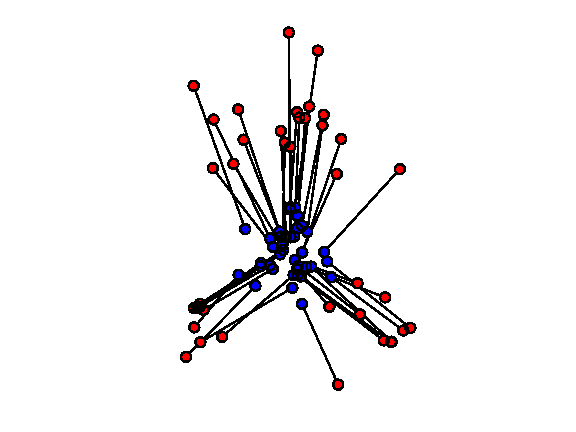# Optimal Transport with Linear Programming¶

Important: Please read the installation page for details about how to install the toolboxes. $\newcommand{\dotp}{\langle #1, #2 \rangle}$ $\newcommand{\enscond}{\lbrace #1, #2 \rbrace}$ $\newcommand{\pd}{ \frac{ \partial #1}{\partial #2} }$ $\newcommand{\umin}{\underset{#1}{\min}\;}$ $\newcommand{\umax}{\underset{#1}{\max}\;}$ $\newcommand{\umin}{\underset{#1}{\min}\;}$ $\newcommand{\uargmin}{\underset{#1}{argmin}\;}$ $\newcommand{\norm}{\|#1\|}$ $\newcommand{\abs}{\left|#1\right|}$ $\newcommand{\choice}{ \left\{ \begin{array}{l} #1 \end{array} \right. }$ $\newcommand{\pa}{\left(#1\right)}$ $\newcommand{\diag}{{diag}\left( #1 \right)}$ $\newcommand{\qandq}{\quad\text{and}\quad}$ $\newcommand{\qwhereq}{\quad\text{where}\quad}$ $\newcommand{\qifq}{ \quad \text{if} \quad }$ $\newcommand{\qarrq}{ \quad \Longrightarrow \quad }$ $\newcommand{\ZZ}{\mathbb{Z}}$ $\newcommand{\CC}{\mathbb{C}}$ $\newcommand{\RR}{\mathbb{R}}$ $\newcommand{\EE}{\mathbb{E}}$ $\newcommand{\Zz}{\mathcal{Z}}$ $\newcommand{\Ww}{\mathcal{W}}$ $\newcommand{\Vv}{\mathcal{V}}$ $\newcommand{\Nn}{\mathcal{N}}$ $\newcommand{\NN}{\mathcal{N}}$ $\newcommand{\Hh}{\mathcal{H}}$ $\newcommand{\Bb}{\mathcal{B}}$ $\newcommand{\Ee}{\mathcal{E}}$ $\newcommand{\Cc}{\mathcal{C}}$ $\newcommand{\Gg}{\mathcal{G}}$ $\newcommand{\Ss}{\mathcal{S}}$ $\newcommand{\Pp}{\mathcal{P}}$ $\newcommand{\Ff}{\mathcal{F}}$ $\newcommand{\Xx}{\mathcal{X}}$ $\newcommand{\Mm}{\mathcal{M}}$ $\newcommand{\Ii}{\mathcal{I}}$ $\newcommand{\Dd}{\mathcal{D}}$ $\newcommand{\Ll}{\mathcal{L}}$ $\newcommand{\Tt}{\mathcal{T}}$ $\newcommand{\si}{\sigma}$ $\newcommand{\al}{\alpha}$ $\newcommand{\la}{\lambda}$ $\newcommand{\ga}{\gamma}$ $\newcommand{\Ga}{\Gamma}$ $\newcommand{\La}{\Lambda}$ $\newcommand{\si}{\sigma}$ $\newcommand{\Si}{\Sigma}$ $\newcommand{\be}{\beta}$ $\newcommand{\de}{\delta}$ $\newcommand{\De}{\Delta}$ $\newcommand{\phi}{\varphi}$ $\newcommand{\th}{\theta}$ $\newcommand{\om}{\omega}$ $\newcommand{\Om}{\Omega}$

This numerical tours details how to solve the discrete optimal transport problem (in the case of measures that are sums of Diracs) using linear programming.

In :
addpath('toolbox_signal')


## Optimal Transport of Discrete Distribution¶

We consider two dicretes distributions $$\forall k=0,1, \quad \mu_k = \sum_{i=1}^{n_k} p_{k,i} \de_{x_{k,i}}$$ where $n_0,n_1$ are the number of points, $\de_x$ is the Dirac at location $x \in \RR^d$, and $X_k = ( x_{k,i} )_{i=1}^{n_i} \subset \RR^d$ for $k=0,1$ are two point clouds.

We define the set of couplings between $\mu_0,\mu_1$ as $$\Pp = \enscond{ (\ga_{i,j})_{i,j} \in (\RR^+)^{n_0 \times n_1} }{ \forall i, \sum_j \ga_{i,j} = p_{0,i}, \: \forall j, \sum_i \ga_{i,j} = p_{1,j} }$$

The Kantorovitch formulation of the optimal transport reads $$\ga^\star \in \uargmin{\ga \in \Pp} \sum_{i,j} \ga_{i,j} C_{i,j}$$ where $C_{i,j} \geq 0$ is the cost of moving some mass from $x_{0,i}$ to $x_{1,j}$.

The optimal coupling $\ga^\star$ can be shown to be a sparse matrix with less than $n_0+n_1-1$ non zero entries. An entry $\ga_{i,j}^\star \neq 0$ should be understood as a link between $x_{0,i}$ and $x_{1,j}$ where an amount of mass equal to $\ga_{i,j}^\star$ is transfered.

In the following, we concentrate on the $L^2$ Wasserstein distance. $$C_{i,j}=\norm{x_{0,i}-x_{1,j}}^2.$$

The $L^2$ Wasserstein distance is then defined as $$W_2(\mu_0,\mu_1)^2 = \sum_{i,j} \ga_{i,j}^\star C_{i,j}.$$

The coupling constraint $$\forall i, \sum_j \ga_{i,j} = p_{0,i}, \: \forall j, \sum_i \ga_{i,j} = p_{1,j}$$ can be expressed in matrix form as $$\Sigma(n_0,n_1) \ga = [p_0;p_1]$$ where $\Sigma(n_0,n_1) \in \RR^{ (n_0+n_1) \times (n_0 n_1) }$.

In :
flat = @(x)x(:);
Cols = @(n0,n1)sparse( flat(repmat(1:n1, [n0 1])), ...
flat(reshape(1:n0*n1,n0,n1) ), ...
ones(n0*n1,1) );
Rows = @(n0,n1)sparse( flat(repmat(1:n0, [n1 1])), ...
flat(reshape(1:n0*n1,n0,n1)' ), ...
ones(n0*n1,1) );
Sigma = @(n0,n1)[Rows(n0,n1);Cols(n0,n1)];


We use a simplex algorithm to compute the optimal transport coupling $\ga^\star$.

In :
maxit = 1e4; tol = 1e-9;
otransp = @(C,p0,p1)reshape( perform_linprog( ...
Sigma(length(p0),length(p1)), ...
[p0(:);p1(:)], C(:), 0, maxit, tol), [length(p0) length(p1)] );


Dimensions $n_0, n_1$ of the clouds.

In :
n0 = 60;
n1 = 80;


Compute a first point cloud $X_0$ that is Gaussian. and a second point cloud $X_1$ that is Gaussian mixture.

In :
gauss = @(q,a,c)a*randn(2,q)+repmat(c(:), [1 q]);
X0 = randn(2,n0)*.3;
X1 = [gauss(n1/2,.5, [0 1.6]) gauss(n1/4,.3, [-1 -1]) gauss(n1/4,.3, [1 -1])];


Density weights $p_0, p_1$.

In :
normalize = @(a)a/sum(a(:));
p0 = normalize(rand(n0,1));
p1 = normalize(rand(n1,1));


Shortcut for display.

In :
myplot = @(x,y,ms,col)plot(x,y, 'o', 'MarkerSize', ms, 'MarkerEdgeColor', 'k', 'MarkerFaceColor', col, 'LineWidth', 2);


Display the point clouds. The size of each dot is proportional to its probability density weight.

In :
clf; hold on;
for i=1:length(p0)
myplot(X0(1,i), X0(2,i), p0(i)*length(p0)*10, 'b');
end
for i=1:length(p1)
myplot(X1(1,i), X1(2,i), p1(i)*length(p1)*10, 'r');
end
axis([min(X1(1,:)) max(X1(1,:)) min(X1(2,:)) max(X1(2,:))]); axis off;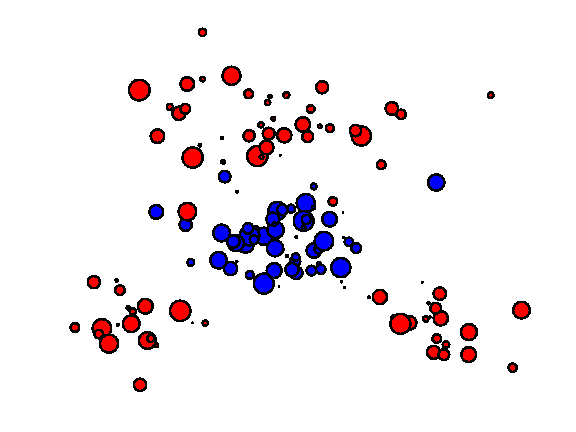Compute the weight matrix $(C_{i,j})_{i,j}.$

In :
C = repmat( sum(X0.^2)', [1 n1] ) + ...
repmat( sum(X1.^2), [n0 1] ) - 2*X0'*X1;


Compute the optimal transport plan.

In :
gamma = otransp(C,p0,p1);


Check that the number of non-zero entries in $\ga^\star$ is $n_0+n_1-1$.

In :
fprintf('Number of non-zero: %d (n0+n1-1=%d)\n', full(sum(gamma(:)~=0)), n0+n1-1);

Number of non-zero: 139 (n0+n1-1=139)


Check that the solution satifies the constraints $\ga \in \Cc$.

In :
fprintf('Constraints deviation (should be 0): %.2e, %.2e.\n', norm(sum(gamma,2)-p0(:)),  norm(sum(gamma,1)'-p1(:)));

Constraints deviation (should be 0): 5.38e-16, 1.01e-17.


## Displacement Interpolation¶

For any $t \in [0,1]$, one can define a distribution $\mu_t$ such that $t \mapsto \mu_t$ defines a geodesic for the Wasserstein metric.

Since the $W_2$ distance is a geodesic distance, this geodesic path solves the following variational problem $$\mu_t = \uargmin{\mu} (1-t)W_2(\mu_0,\mu)^2 + t W_2(\mu_1,\mu)^2.$$ This can be understood as a generalization of the usual Euclidean barycenter to barycenter of distribution. Indeed, in the case that $\mu_k = \de_{x_k}$, one has $\mu_t=\de_{x_t}$ where $x_t = (1-t)x_0+t x_1$.

Once the optimal coupling $\ga^\star$ has been computed, the interpolated distribution is obtained as $$\mu_t = \sum_{i,j} \ga^\star_{i,j} \de_{(1-t)x_{0,i} + t x_{1,j}}.$$

Find the $i,j$ with non-zero $\ga_{i,j}^\star$.

In :
[I,J,gammaij] = find(gamma);


Display the evolution of $\mu_t$ for a varying value of $t \in [0,1]$.

In :
clf;
tlist = linspace(0,1,6);
for i=1:length(tlist)
t=tlist(i);
Xt = (1-t)*X0(:,I) + t*X1(:,J);
subplot(2,3,i);
hold on;
for i=1:length(gammaij)
myplot(Xt(1,i), Xt(2,i), gammaij(i)*length(gammaij)*6, [t 0 1-t]);
end
title(['t=' num2str(t,2)]);
axis([min(X1(1,:)) max(X1(1,:)) min(X1(2,:)) max(X1(2,:))]); axis off;
end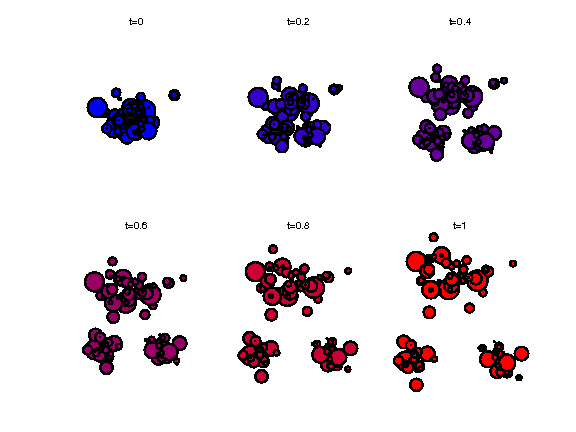## Optimal Assignement¶

In the case where the weights $p_{0,i}=1/n, p_{1,i}=1/n$ (where $n_0=n_1=n$) are constants, one can show that the optimal transport coupling is actually a permutation matrix. This properties comes from the fact that the extremal point of the polytope $\Cc$ are permutation matrices.

This means that there exists an optimal permutation $\si^\star \in \Sigma_n$ such that $$\ga^\star_{i,j} = \choice{ 1 \qifq j=\si^\star(i), \\ 0 \quad\text{otherwise}. }$$ where $\Si_n$ is the set of permutation (bijections) of $\{1,\ldots,n\}$.

This permutation thus solves the so-called optimal assignement problem $$\si^\star \in \uargmin{\si \in \Sigma_n} \sum_{i} C_{i,\si(j)}.$$

Same number of points.

In :
n0 = 40;
n1 = n0;


Compute points clouds.

In :
X0 = randn(2,n0)*.3;
X1 = [gauss(n1/2,.5, [0 1.6]) gauss(n1/4,.3, [-1 -1]) gauss(n1/4,.3, [1 -1])];


Constant distributions.

In :
p0 = ones(n0,1)/n0;
p1 = ones(n1,1)/n1;


Compute the weight matrix $(C_{i,j})_{i,j}.$

In :
C = repmat( sum(X0.^2)', [1 n1] ) + ...
repmat( sum(X1.^2), [n0 1] ) - 2*X0'*X1;


Display the coulds.

In :
clf; hold on;
myplot(X0(1,:), X0(2,:), 10, 'b');
myplot(X1(1,:), X1(2,:), 10, 'r');
axis equal; axis off;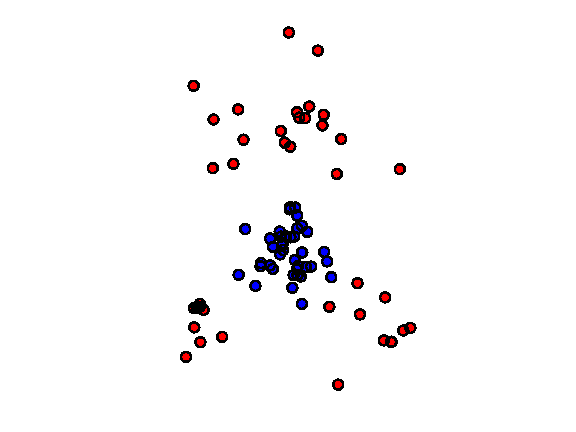Solve the optimal transport.

In :
gamma = otransp(C,p0,p1);


Show that $\ga$ is a binary permutation matrix.

In :
clf;
imageplot(gamma);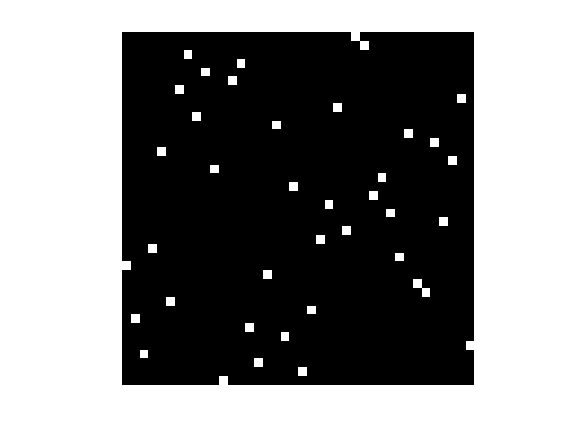Display the optimal assignement.

In :
clf; hold on;
[I,J,~] = find(gamma);
for k=1:length(I)
h = plot( [X0(1,I(k)) X1(1,J(k))], [X0(2,I(k)) X1(2,J(k))], 'k' );
set(h, 'LineWidth', 2);
end
myplot(X0(1,:), X0(2,:), 10, 'b');
myplot(X1(1,:), X1(2,:), 10, 'r');
axis equal; axis off;